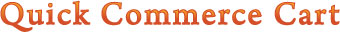# Shopping Cart Software

• Field 8: itemweight (optional, may be required by itemshipmethod) -- This field contains the item weight. In order to use approved shipping standards, all products using itemshipmethod must have their weight listed in pounds.

Weight conversion table
 Ounces Pounds 1 = 0.0625 2 = 0.1250 3 = 0.1875 4 = 0.2500 5 = 0.3125 6 = 0.3750 7 = 0.4375 8 = 0.5000 9 = 0.5625 10 = 0.6250 11 = 0.6875 12 = 0.7500 13 = 0.8125 14 = 0.8750 15 = 0.9375 16 = 1.0000

Most merchants will only need to enter weights here. However, some may need to add box dimensions. This can be done using this format:

QTY#WEIGHT:LENGTH:WIDTH:HEIGHT

For example if you have a 6 pound box that measures 10 inches by 8 inches by 4 inches you would enter:

6:10:8:4

You can specify multiple boxes in an item like so:

WEIGHT:LENGTH:WIDTH:HEIGHT=WEIGHT:LENGTH:WIDTH:HEIGHT=WEIGHT:LENGTH:WIDTH:HEIGHT

So if you have the original box above as well as a 3 pound box measuring 5 inches by 3 inches by 1 inch you enter

6:10:8:4=3:5:3:1

Note: If you do not specify a length, width, and height then the shopping cart will use an internal algorithm to guess the dimensions. If you require extremely accurate shipping charges then you MUST enter box dimensions.